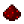# bit.bxorFunction bit.bxor Computes the bitwise exclusive OR of two numbers Syntax bit.bxor(number m, number n) Returns number the value of m XOR n Part of ComputerCraft API bit

## ExamplesExample XOR the number 18 (10010) with the number 3 (00011), yielding 17 (10001) Code ```print(bit.bxor(18, 3)) ``` Output 17

## Explanation

All bit operations operate in binary numeral system . An exclusive OR operation between two bits yields a 1 if the bits are unequal and a 0 if they are equal. This function produces an output by computing the XOR of each bit of its two inputs independently. So, for the example above:

 Bit index: Input 1 (18): Input 2 (3): Calculation: Output (17): 4 3 2 1 0 1 0 0 1 0 0 0 0 1 1 1 ≠ 0 0 = 0 0 = 0 1 = 1 0 ≠ 1 1 0 0 0 1